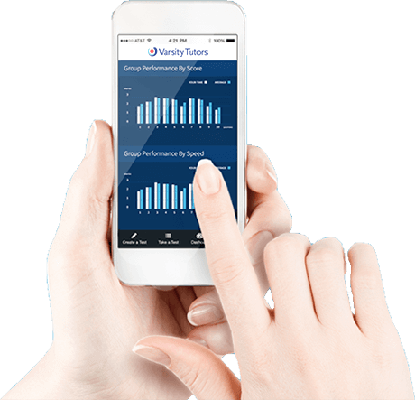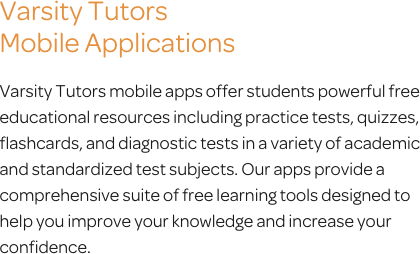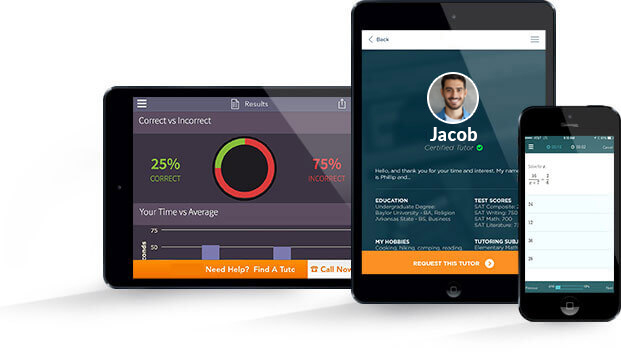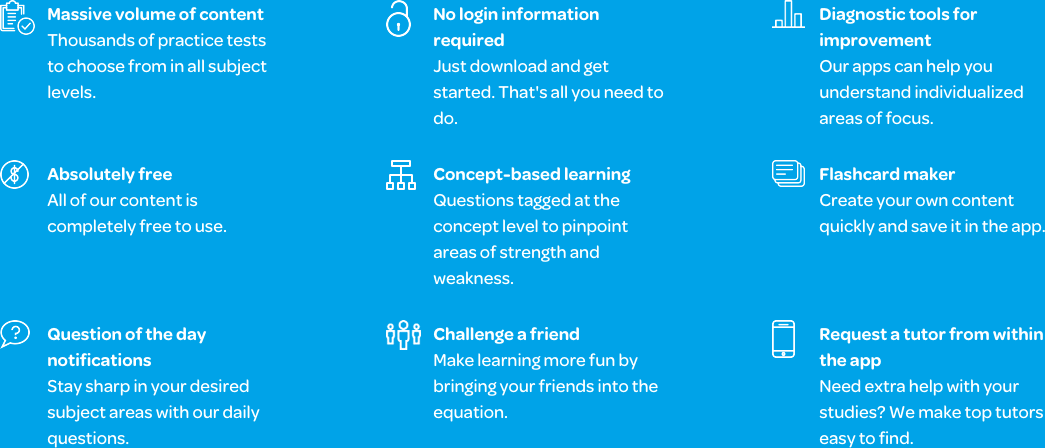# Varsity Tutors Linear Algebra mobile app### The Varsity Tutors Linear Algebra Mobile App

If you are a college student who is studying Linear Algebra, and you’re finding yourself struggling with the course content or needing to create a study session to prepare for an upcoming exam, Varsity Tutors’ Learning Tools can help you get the assistance needed to succeed. By downloading the Varsity Tutors Linear Algebra mobile app, you can turn your iOS or Android device into a portable study session.

This program is an excellent way to help you prepare for test day, and it gives you access to a number of helpful resources. For example, if you want to learn a new concept each day, consider utilizing the Question of the Day. By doing so, you can answer a question related to Linear Algebra, such as matrices, linear equations, or anything else covered in the content. On the other hand, if an in-depth study routine is what you want, then consider studying with the Learn by Concept feature. Where the Question of the Day helps you get a short study session, Learn by Concept provides you with an interactive syllabus that explains all of the concepts in Linear Algebra. Think of it as a textbook for your device, where you can find anything you want to study, like linear mapping, the identity matrix, or even gradients and hessians.

After you have reviewed or learned a sufficient amount of material, consider reinforcing your knowledge using the flashcards. They are easy to use and have each component of Linear Algebra arranged by topic. You can even create your own deck, thus helping you really focus on drilling those confusing and difficult areas of the subject. Furthermore, every problem presented through the flashcards comes with important information, like formulas and definitions.

Once you feel that you have gotten the revision needed to succeed, try testing your knowledge with one of the program’s practice tests. There are a wide range of short practice tests that are arranged by topics, and they are a great way to test your understanding of a specific area in Linear Algebra. If you want to evaluate your overall understanding of the subject, you can take one of the Full-Length Practice Tests. These practice tests are much longer than their counterparts and cover all of the material that will be present on an upcoming exam. Furthermore, since they are designed to emulate the actual test itself, you will be able to mentally prepare for test day while you study.

One of the best features of the practice tests are the answer keys, which come with detailed explanations of every problem that tell you how the correct answer was reached. You can also see your results in graph format, look at how much time you spent answering each question, and compare your results to the average test taker.

The Varsity Tutors Linear Algebra mobile learning app is available through iTunes and the Google Play Store. By adding it to your study plan, you are able to supplement your learning experience and get the skills and preparation to help you succeed in the course.Linear algebra is a course you may intend to take if you are seeking a future involving math, engineering or science. In linear algebra, you will study how the line sets of equations can transform into different dimensions. This field of math can be very difficult and specific, requiring you to use skills that allow you to employ multi-dimensional thinking while you look at information and structures. The course is traditionally taken by students in college after they have completed two terms of calculus. Like many math courses, linear algebra focuses on a number of concepts, each of which gives way to a variety of smaller topics that you need to master in order to succeed. This may sound intense, but you can review everything with the Linear Algebra app from Varsity Tutors, available for any iPhone, iPad, or Android-powered device.

Linear algebra allows you to take the concepts you learned in basic math courses, as well as previous advanced classes, and apply them to linear maps and vector spaces in a way that permits you to think beyond the abstract. Due to the numerous applications of vectors and matrices, there are many fields of study and career you can dive into with a background in linear algebra. Matrices are vital to quantum mechanics, statistics, and special relativity. Though this area of math may be relatively new, it has gained immense value as an application-based, rather than theory-based, field.

The study of linear algebra is intensive. You will be required to master each concept thoroughly, as it will ensure that you can progress further into more advanced coursework. Linear equations allow you to solve the problems you will face in your linear algebra class. You must know the specific formulas necessary to calculate the determinant of a matrix equation, which can allow you to identify a unique or consistent solution. You need to be capable of identifying a homogeneous case and a non-homogeneous case, each of which require a vastly different strategy in order to successfully solve. Further, you will learn about the echelon and row echelon forms, and how the Gaussian elimination plays a role in certain matrix shaping. You have to understand the reasoning behind the different forms of matrices. In addition, you will learn how to use the row of a matrix to solve a system or identify the inverse. You will learn about the row equivalent, which requires one of two row operations to identify. All of the topics of the course are covered in-depth by the Varsity Tutors Linear Algebra app, available for free download from iTunes or the Google Play Store.

As the primary structure of linear algebra, vector spaces are of vital importance. You need to be capable of identifying and forming vectors, while understanding the specialized vocabulary terms that note its elements. There are two operations you need to master: scalar multiplication and vector addition. Each of these can be applied to an axiom depending on the axiom’s type and significance. During this area, you must have a strong understanding of previous content, such as distribution, inverses, and associative properties. As you delve into the coursework, you will learn how to differentiate from various axioms. You will learn how to multiply matrices together and with a vector, as well as how to multiply two vectors together.

When you enter the matrix calculus period of your coursework, you will be faced with a number of core topics that you need to become proficient in. You will learn about eigenvalues, which are relatively new to the field of linear algebra. With eigenvalues, you also delve into eigenvectors, which provide a unique way of looking at a matrix. Due to the complexities presented in linear algebra, you will need to develop strategies that allow you to quickly identify types of structures. To accomplish this, you can find the characteristic vector by solving for the invariant sets, and later, the identity matrix. Furthermore, you will learn how to transform a matrix into a diagonalizable matrix to solve them.

You will spend a good deal of time working with linear and quadratic functions. You need to understand the Hessian matrix and gradient, and how they are applied to these types of functions. By using a few simple strategies, you can become proficient in determining if something is a Hessian matrix. When you have a grasp of gradients, you can quickly identify the components that make up a function. The gradient provides an improved method to represent several rates of change within a single vector. Further, your class will spend a period emphasizing least squares. You must be capable of finding the least squares solution, which ensures you use as little space as possible while solving a problem.

There are numerous types of operations and properties you will need to develop an understanding of in the next portion of linear algebra, all of which you can study with the free Varsity Tutors Linear Algebra app for iPhones, iPads, and Android-powered devices. Your teacher will delve more specifically into eigenvalues and eigenvectors. There are many ways that these can be applied to math. They are necessary in order for you to understand the methods used to test subspaces. To get an understanding of these concepts, you must become proficient in identifying an exception vector, or eigenvector, and use it to solve for eigenvalues, which are used to note rates of change in a vector. You will learn how to apply these formulas to symmetric matrices, as well as how to determine if a set of vectors is linearly independent or linearly dependent. Each are imperative concepts for you to completely understand dimensions. With these concepts understood, you will be able to calculate the set of vectors in a space.

With a solid handle on the above concepts, you can begin learning linear mapping, which is a critical component to dimensional computation. Linear mapping allows you to track linear transformations as they occur between vector spaces. It is the alternative method of testing the space between vectors, and is vastly unique in comparison to using eigenvalues and eigenvectors. You should be capable of determining the ideal strategies based on the situation presented. You will learn about the norm, how it functions, and how it is important to your coursework. In addition, you’ll learn to work with orthogonal vectors and subspaces, as well as null spaces and how each of these correlate to the matrices you are working with. Finally, you will develop the skills necessary to solve for the determinant of matrices, as well as the diagonal and identity.

Linear algebra is a thorough course that allows you to develop the skills and understanding necessary to delve into more advanced topics in your future coursework. Start building these skills today by downloading Varsity Tutors’ free Linear Algebra app from iTunes or the Google Play Store.